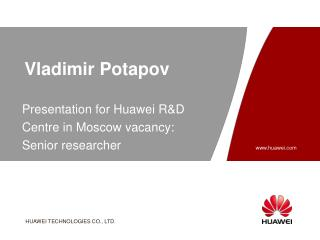DownloadDownload PresentationDownload Presentation- - - - - - - - - - - - - - - - - - - - - - - - - - - E N D - - - - - - - - - - - - - - - - - - - - - - - - - - -
##### Presentation Transcript

1. Vladimir Potapov Presentation for Huawei R&D Centre in Moscow vacancy: Senior researcher

2. Education • M.Sc. in Mathematics, Novosibirsk State University, 1994 • Ph.D. in Mathematics, Sobolev Institute of Mathematics, 1997

3. Career/ Employment • 1994-present – NSU, Department of Mathematical Analysis at the Mechanics and Mathematics Division, 1994-1997 - post graduate student, 1997-2001 - teacher (assistant professor), 2001-present – docent (associate professor). • 1998-present – Sobolev Institute of Mathematics, Laboratory of Discrete Analysis, senior researcher

4. Areas of research • Information Theory coding theory, source coding, cryptography • Combinatorics • Bioinformatics • Approximation Theory

5. Research experience • Grants of Russian Foundation of Basic Research, 95-01-01343, 99-01-00531, 02-01-00939, 05-01-00364, 08-01-00671, 10-01-00424, 10-01-00616, 11-01-997 • The author of main scientific results of SIM in 2001, 2003, 2006, 2011, 2012 • Courses taught Mathematical analysis; Functional Analysis; Linear alge-bra and geometry; Discrete Mathematics; Information theory at NSU, Perfect combinatorial structures; Information theory and Coding at the Summer student's school ‘Modern Mathematics’

6. Selective publications • R.E.Krichevskii, V.N.Potapov. Compression and restoration of square intergable function // Electronic Research Announcements of the American Mathematical Society. 1996. V. 2, N 1. P. 42-49.  • V.N.Potapov. ε-Entropy of compact sets in C, and tabulation of continuous functions // Siberian Math. J. 1997.V.38,N 4. P.759–771.  • Y.L.Orlov, V.P.Filippov, V.N.Potapov, N. A.Kolchanov. Construction of stochastic context trees for genetic texts // In Silico Biology. 2002. v. 2, N. 3. P. 233-247. • Y.L.Orlov, V.N.Potapov. Complexity: an internet resource for analysis of DNA sequence complexity // Nucl. Acids. Res. 2004. V. 32. P. 628-633.

7. Selective publications Potapov V.N. Information Theory. Source Coding. NSU, Novosibirsk. 1999. 71 pp. (in Russian) R.E.Krichevskii, V.N.Potapov. Encoding of run lengths and pyramid cubic lattices // IEEE Trans. Inform. Theory. 1999. V.5, N. 4. P. 1347-1350. V.N.Potapov. Redundancy estimates for the Lempel-Ziv algorithm of data compression // Discrete Appl. Math. 2004. V.35, N 1-3. P. 245—254. V. N. Potapov. A survey of methods for the undistorted coding of discrete sources //Diskretn. Anal. Issled. Oper., Ser. 1. 1999.6:4, P. 49–91. (in Russian) Page 7

8. Selective publications D.S.Krotov, V.N.Potapov. On multifold MDS and perfect codes that are not splittable into onefold codes // Problems of Information Transmission. 2004. V. 40, N 1. С. 5--12. V.N.Potapov. A lower bound for the number of transitive perfect codes // Journal of Applied and Industrial Mathematics. 2007. V.1, N 3. P. 373-379. D.S.Krotov, V.N.Potapov. Switching equivalence of n-ary quasigroups of order 4 and perfect binary codes // Problems of Information Transmission. 2010. V. 46, N 3. P. 219-224. V.N.Potapov. Cardinality spectra of components of correlation immune functions, bent functions, perfect colorings, and codes // Problems of Information Transmission. 2012. V.48, N1. P. 47--55. Page 8

9. Selective publications D.S.Krotov, V.N.Potapov. n-Ary quasigroups of order 4 // SIAM J. Discrete Math. 2009. V. 23, N 2. P. 561-570. D.S.Krotov, V.N.Potapov. On connection between reducibility on an n-ary quasigroup and that of its retracts // Discrete Math. 2011. V. 311, N 1. P.58-66. V.N.Potapov. On extensions of partial n-quasigroups of order 4 // Siberian Advances in Mathematics. 2012. V.22, N 2. P.135-151. V.N.Potapov, D.S.Krotov. On the number of n-ary quasigroups of finite order // Discrete Mathematics and Applications. 2011. V. 21, N 5-6. P. 575-585. Page 9

10. My proposals • I'm going to solve applied mathematical problems • Preferred research directions error-correcting codes discrete functions for symmetric-key cryptography data compression and transmission